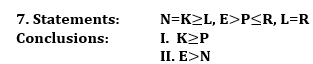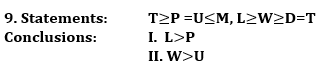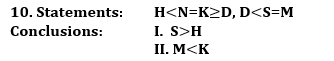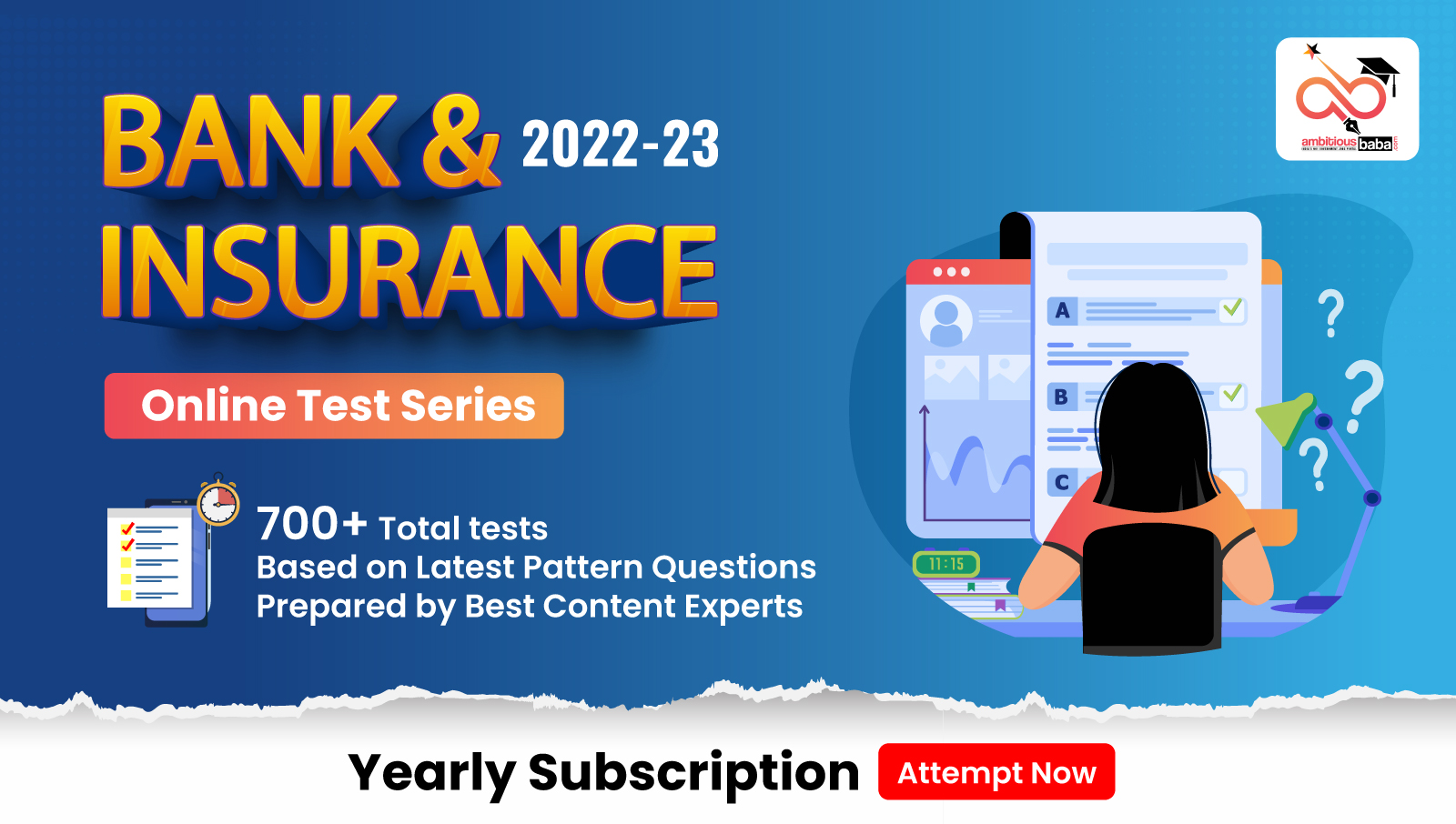# IBPS PO Pre Reasoning Ability Quiz – 13

## IBPS PO Pre Reasoning Ability Quiz

Reasoning Ability plays a very crucial role in every competitive examination. With consistent practice, candidates can ace this section in examination. In this article, we bring to you IBPS PO Reasoning Ability Quiz to boost your preparation. This IBPS PO Reasoning Ability Quiz contains various types of questions ranging from easy to difficult level. This IBPS PO Reasoning Ability Quiz is absolutely FREE. Candidates will be provided with a detailed explanation of each question in this IBPS PO Reasoning Ability Quiz. Question given in this IBPS PO Reasoning Ability Quiz are based on the latest or updated exam pattern. In order to be able to answer questions quickly and efficiently in upcoming exams, aspirants must practice this IBPS PO Reasoning Ability Quiz.

Directions (1-5): These questions are based on the following information.
In a certain code:
improves circulation cardiovascular health – f3 b7 n4 j22
cold showers treat ailments – i14 p2 s10 j4
keeps skin hair healthy- l2 b2 f10 f14
strengthens immunity increases testosterone- o18 u22 f6 n4
increases energy and vitality- o3 o6 j4 o18

1. What will be the code for showers?
(a) i14
(b) s6
(c) s10
(d) h14
(e) None of these

Ans. a
Exp. The given alphabets are coded as per following pattern:
(i) First letter of the code represents the next letter of the second letter of the given word.
(ii) If the total number of letters in the code is even, then the number in the code represents the -(Total number of letters in the given alphabet)/2.
(iii) If the total number of letters in the code is odd, then the number in the code represents the -(Total number of letters in the given alphabet)x2

2. What must be the code for treaty?
(a) Can’t be determined
(b) s10
(c) s3
(d) v3
(e) z10

Ans. c
Exp. The given alphabets are coded as per following pattern:
(i) First letter of the code represents the next letter of the second letter of the given word.
(ii) If the total number of letters in the code is even, then the number in the code represents the -(Total number of letters in the given alphabet)/2.
(iii) If the total number of letters in the code is odd, then the number in the code represents the -(Total number of letters in the given alphabet)x2

3. Which of the following can be coded as improvements?
(a) J12
(b) n12
(c) m4
(d) n6
(e) m6

Ans. d
Exp. The given alphabets are coded as per following pattern:
(i) First letter of the code represents the next letter of the second letter of the given word.
(ii) If the total number of letters in the code is even, then the number in the code represents the -(Total number of letters in the given alphabet)/2.
(iii) If the total number of letters in the code is odd, then the number in the code represents the -(Total number of letters in the given alphabet)x2

4. If all the letters of the word “ailments” are reversed then what would be the code for the resulting letter?
(a) j4
(b) u4
(c) t8
(d) t4
(e) None of these

Ans. b
Exp. The given alphabets are coded as per following pattern:
(i) First letter of the code represents the next letter of the second letter of the given word.
(ii) If the total number of letters in the code is even, then the number in the code represents the -(Total number of letters in the given alphabet)/2.
(iii) If the total number of letters in the code is odd, then the number in the code represents the -(Total number of letters in the given alphabet)x2

5. What could be the code for go?
(a) p2
(b) h1
(c) h2
(d) p1
(e) l1

Ans. d
Exp. The given alphabets are coded as per following pattern:
(i) First letter of the code represents the next letter of the second letter of the given word.
(ii) If the total number of letters in the code is even, then the number in the code represents the -(Total number of letters in the given alphabet)/2.
(iii) If the total number of letters in the code is odd, then the number in the code represents the -(Total number of letters in the given alphabet)x2

Directions (6-10): In these questions, a relationship between different elements is shown in the statements. The statements are followed by two conclusions. Give answer.(a) if only conclusion I is true.
(b) if only conclusion II is true.
(c) if either conclusion I or II is true.
(d) if neither conclusion I nor II is true.
(e) if both conclusions I and II are true.

Ans. b(a) if only conclusion I is true.
(b) if only conclusion II is true.
(c) if either conclusion I or II is true.
(d) if neither conclusion I nor II is true.
(e) if both conclusions I and II are true.

Ans. a(a) if only conclusion I is true.
(b) if only conclusion II is true.
(c) if either conclusion I or II is true.
(d) if neither conclusion I nor II is true.
(e) if both conclusions I and II are true.

Ans. d(a) if only conclusion I is true.
(b) if only conclusion II is true.
(c) if either conclusion I or II is true.
(d) if neither conclusion I nor II is true.
(e) if both conclusions I and II are true.

Ans. d(a) if only conclusion I is true.
(b) if only conclusion II is true.
(c) if either conclusion I or II is true.
(d) if neither conclusion I nor II is true.
(e) if both conclusions I and II are true.

Ans. d

###Click to Buy Bank MahaCombo Package

Recommended PDF’s for:

#### Most important PDF’s for Bank, SSC, Railway and Other Government Exam : Download PDF Now

AATMA-NIRBHAR Series- Static GK/Awareness Practice Ebook PDF Get PDF here
The Banking Awareness 500 MCQs E-book| Bilingual (Hindi + English) Get PDF here
AATMA-NIRBHAR Series- Banking Awareness Practice Ebook PDF Get PDF here
Computer Awareness Capsule 2.O Get PDF here
AATMA-NIRBHAR Series Quantitative Aptitude Topic-Wise PDF Get PDF here
AATMA-NIRBHAR Series Reasoning Topic-Wise PDF Get PDF Here
Memory Based Puzzle E-book | 2016-19 Exams Covered Get PDF here
Caselet Data Interpretation 200 Questions Get PDF here
Puzzle & Seating Arrangement E-Book for BANK PO MAINS (Vol-1) Get PDF here
ARITHMETIC DATA INTERPRETATION 2.O E-book Get PDF here

3